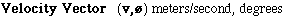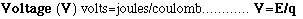# Hands-On-Physics

### Variables

Variables are names for quantites. These names appear in mathematical expressions as letters. For example; in the expression y = 7x, both y and x are variables. Once the variable x is assigned a quantity the value of y is fixed. In this example, when x is set to 2, y becomes fixed at 14.

Dependent Variable:
When two variables are related, we say that one depends on the other. This variable is called the dependent variable. The"other" variable is free to roam so it is called the independent variable. In a scientific experiment, the experimenter chooses values for the independent variable, runs the experiment, and measures the dependent variable. Ordered pairs of choosen and measured values (independent and dependent) are often plotted on a two dimensional graph for visualization. The dependent variable is traditionally plotted on the vertical axis.

Independent variable:
When two variables are related, one of the variable is free to roam so it is called the independent variable. The other variable depends on the first, so it gets the name dependent. In a scientific experiment, the experimenter chooses values for the independent variable, runs the experiment, and then measures the dependent variable. Ordered pairs of choosen and measured values (independent and dependent) are often plotted on a two dimensional graph for visualization. The independent variable is traditionally plotted on the horizontal axis.The "length" of the velocity vector is the speed. The direction of the motion (ø), is also part of the velocity vector.Voltage is an energy measure, the energy carried by one coulomb of electrical charge. The voltage between two points in a circuit is the amount of energy available for pushing each coulomb of charge from one of these points to the other.Work is done on an object whenever it moves because it is pushed or pulled. Work increases the energy of the object.

A | B | C | D | E | F | G | H | I | J | K | L | M | N
O | P | Q | R | S | T | U | V | W | X | Y | Z
Top of Glossary